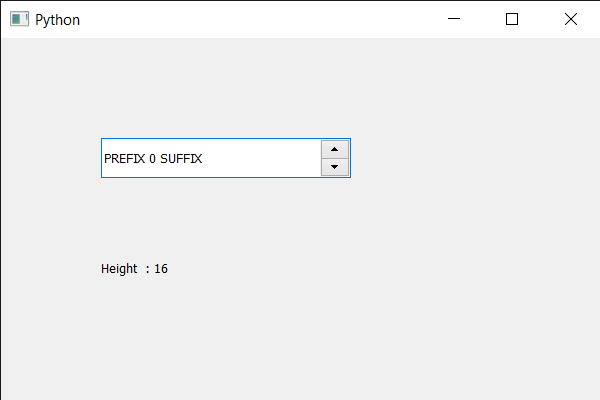# PyQt5 QSpinBox – Getting height of font

In this article we will see how we can get the height of the spin box’s font i.e its text. Height is the vertical length of the text. Height of font is always equal to ascent +descent +1 (the 1 is for the base line).

In order to do this we use `height` method with the spin box’s QFontMetrics object.

Syntax : font_metrics.height()

Argument : It takes no argument

Return : It returns integer.

Below is the implementation

 `# importing libraries ` `from` `PyQt5.QtWidgets ``import` `*`  `from` `PyQt5 ``import` `QtCore, QtGui ` `from` `PyQt5.QtGui ``import` `*`  `from` `PyQt5.QtCore ``import` `*`  `import` `sys ` ` `  ` `  `class` `Window(QMainWindow): ` ` `  `    ``def` `__init__(``self``): ` `        ``super``().__init__() ` ` `  `        ``# setting title ` `        ``self``.setWindowTitle(``"Python "``) ` ` `  `        ``# setting geometry ` `        ``self``.setGeometry(``100``, ``100``, ``600``, ``400``) ` ` `  `        ``# calling method ` `        ``self``.UiComponents() ` ` `  `        ``# showing all the widgets ` `        ``self``.show() ` ` `  `        ``# method for widgets ` `    ``def` `UiComponents(``self``): ` `        ``# creating spin box ` `        ``self``.spin ``=` `QSpinBox(``self``) ` ` `  `        ``# setting geometry to spin box ` `        ``self``.spin.setGeometry(``100``, ``100``, ``250``, ``40``) ` ` `  `        ``# setting range to the spin box ` `        ``self``.spin.setRange(``0``, ``999999``) ` ` `  `        ``# setting prefix to spin ` `        ``self``.spin.setPrefix(``"PREFIX "``) ` ` `  `        ``# setting suffix to spin ` `        ``self``.spin.setSuffix(``" SUFFIX"``) ` ` `  `        ``# creating a label ` `        ``label ``=` `QLabel(``self``) ` ` `  `        ``# making label multi line ` `        ``label.setWordWrap(``True``) ` ` `  `        ``# setting geometry to the label ` `        ``label.setGeometry(``100``, ``200``, ``300``, ``60``) ` ` `  `        ``# getting font metrics ` `        ``f_metrics ``=` `self``.spin.fontMetrics() ` ` `  ` `  `        ``# getting height of the font ` `        ``height ``=` `f_metrics.height() ` ` `  `        ``# setting text to the label ` `        ``label.setText(``"Height  : "` `+` `str``(height)) ` ` `  ` `  `# create pyqt5 app ` `App ``=` `QApplication(sys.argv) ` ` `  `# create the instance of our Window ` `window ``=` `Window() ` ` `  `# start the app ` `sys.exit(App.``exec``()) `

Output :My Personal Notes arrow_drop_upCheck out this Author's contributed articles.

If you like GeeksforGeeks and would like to contribute, you can also write an article using contribute.geeksforgeeks.org or mail your article to contribute@geeksforgeeks.org. See your article appearing on the GeeksforGeeks main page and help other Geeks.

Please Improve this article if you find anything incorrect by clicking on the "Improve Article" button below.

Article Tags :

Be the First to upvote.

Please write to us at contribute@geeksforgeeks.org to report any issue with the above content.